Ch 3. Fluid Kinematics Multimedia Engineering Fluids FlowDescriptions Steady &Unsteady Streamlines,Streaklines Velocity &Acceleration IrrotationalFlow
 Chapter 1. Basics 2. Fluid Statics 3. Kinematics 4. Laws (Integral) 5. Laws (Diff.) 6. Modeling/Similitude 7. Inviscid 8. Viscous 9. External Flow 10. Open-Channel Appendix Basic Math Units Basic Equations Water/Air Tables Sections Search eBooks Dynamics Fluids Math Mechanics Statics Thermodynamics Author(s): Chean Chin Ngo Kurt Gramoll ©Kurt GramollFLUID MECHANICS - THEORY

Two basic and important field variables in the study of fluid mechanics are the velocity and acceleration of the fluid, and they are the focus of the discussion in this section. Both the velocity and acceleration equations are presented in Eulerian viewpoint.

Velocity Field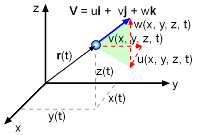Velocity Components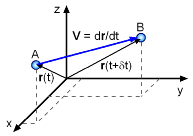Velocity Field

Velocity is an important basic parameter governing a flow field. Other field variables such as the pressure and temperature are all influenced by the velocity of the fluid flow. In general, velocity is a function of both the location and time. The velocity vector can be expressed in Cartesian coordinates as

 V = V(x,y,z,t)      = u(x,y,z,t) i + v(x,y,z,t)j + w (x,y,z,t) k

where the velocity components (u, v and w) are functions of both position and time. That is, u = u(x, y, z, t), v = v(x, y, z, t) and w = w(x, y, z, t). This velocity description is called the velocity field since it describes the velocity of all points, Eulerian viewpoint, in a given volume.

For a single particle, Lagrangian viewpoint, the velocity is derived from the changing position vector, or

V = dr/dt = = dx/dt i + dy/dt j + dz/dt k

where r is the position vector (r = xi + yj + zk). Notice, the velocity is only a function of time since it is only tracking a single particle.

Acceleration Field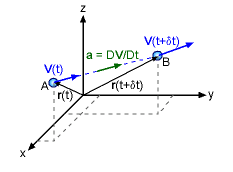Acceleration Field

Another important parameter in the study of fluid in motion is the acceleration. Acceleration is related to the velocity, and it can be determined once the velocity field is known. The acceleration is the change in velocity, δV, over the change in time, δt,

a = [dV(t + δ) - dV(t)] / δt = δV/δt = dV/dt

But it is not just a simple derivative of just time since the velocity is a function time, AND space (x,y,z). The change in velocity must be track in both time and space. Using the chain rule of calculus, the change in velocity is,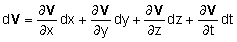orThis can simplified using u, v, and w, the velocity magnitudes in the three coordinate directions. In cartesian coordinates, the acceleration field is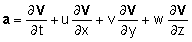This expression can be expanded and rearranged as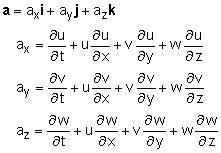The acceleration equation can also be written in polar coordinates and are given in the Basic Equations appendix.

Material Derivative

The time and space derivative used to determine the acceleration field from the velocity is so common in fluid mechanics, it has a special name. It is called the Material or Substantial Derivative and has a special symbol, D( )/Dt. For cartesian coordinates, it is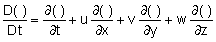or in vector form,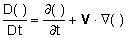where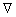is the gradient operator. From the above equation, it can be seen that the material derivative consists of two terms. The first term  ∂t( )/∂t is referred to as the local rate of change, and it represents the effect of unsteadiness. For steady flow, the local derivative vanishes (i.e., ∂t( )/∂t = 0).

The second term,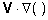, is referred to as the convective rate of change, and it represents the variation due to the change in the position of the fluid particle, as it moves through a field with a gradient. If there is no gradient (no spatial change) then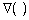is zero so there is no convective change.

As an example, the acceleration field equation can be written as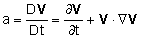Practice Homework and Test problems now available in the 'Eng Fluids' mobile app
Includes over 250 problems with complete detailed solutions.
Available now at the Google Play Store and Apple App Store.